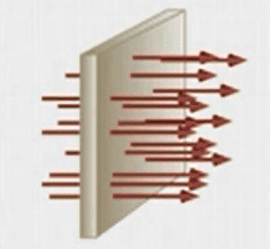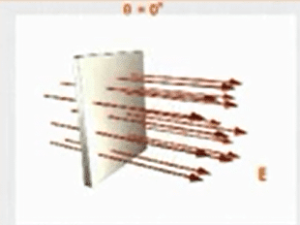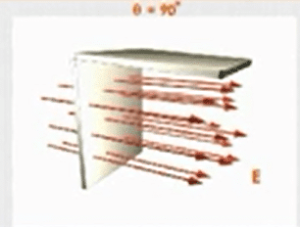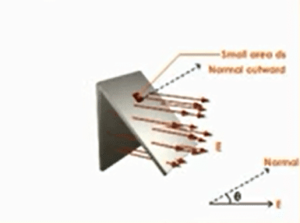Electricity & Megnetism

# Electric Flux formula and Unit

The total number of field lines passing through a certain element of area is called electric flux. It is a scalar quantity. SI Units for electric flux are Nm²/c. Electric flux is positive when the area is held perpendicular to the field lines and negative when the area is held parallel to the field lines. It may also be defined as “The scalar product of electric field intensity and vector area  A“.

## What is the formula of Electric flux?

It is denoted by the Greek letter Φ.

Φ=E.A

Φ=EAcosθ

Where θ is the angle between E and A. It is a scalar quantity.

### Electric Flux SI Unit

Its SI unit Nm²/c.The below figure shows electric flux through a surface normal to E.When we place an element of an area in an electric field, some of the lines of force pass through it. In order to give a quantitative meaning to flux, the field lines are drawn such that the number of field lines passing through a unit area held perpendicular to field lines at a point represents the intensity E of the field at that point. Suppose at a given point the value of E is 4N/C. This means that if 1m² area is held perpendicular to the field lines at this point,4 field lines will pass through it.

### When is Electric Flux Maximum?

Consider a certain areais held perpendicular to the field lines E but the direction of A is parallel to E  as shown in the figure below.In this case, the angle between E and  A is O°

Φ=EAcosθ

Φ=EAcos0°

Φ=EA.1

Φ=EA

### When is electric flux zero?

If the area is held parallel to the field lines E, shown in the figure belowIn this case angle between and A is 90°.
Thus                                                             Φ=EAcosθ

Φ=EAcos90°

We know cos90°=0

Φ=0

Now we consider the case in which A is neither perpendicular nor parallel to field lines but is inclined at angle θ with the lines as shown in figureIn this case, we have to find the projection of area A which is perpendicular to the field lines. The area of this projection  is
Acosθ.The flux Φ in this case is

Φ=EAcosθ=EA

Where θ is the angle between the field lines and normal to the area.